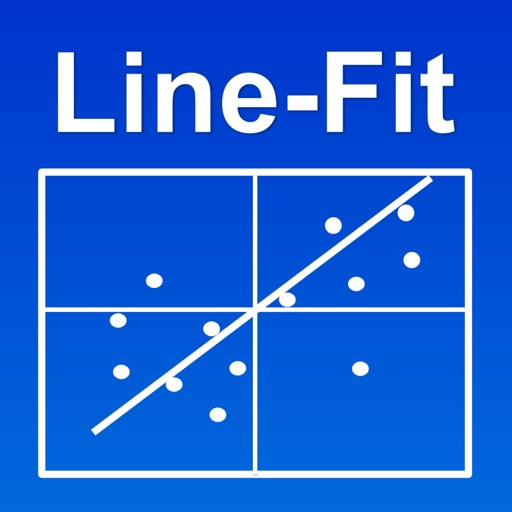## The Line-Fit app uses linear regression to model the relationship between two variables (x and y) by fitting a linear equation to observed data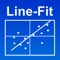# Line-Fit

by Bjarne Berge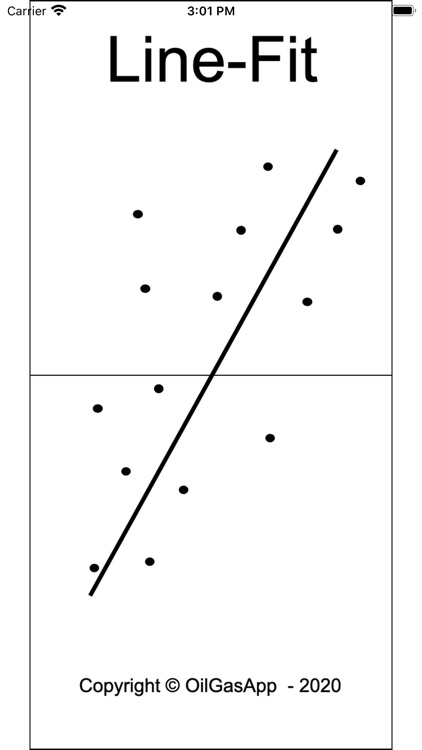The Line-Fit app uses linear regression to model the relationship between two variables (x and y) by fitting a linear equation to observed data. One variable is considered to be an explanatory (independent) variable, and the other is considered to be a response (dependent) variable.### App Details

Version
2.0
Rating
(3)
Size
0Mb
Genre
Productivity Utilities
Last updated
November 15, 2020
Release date
June 14, 2019

### App Screenshots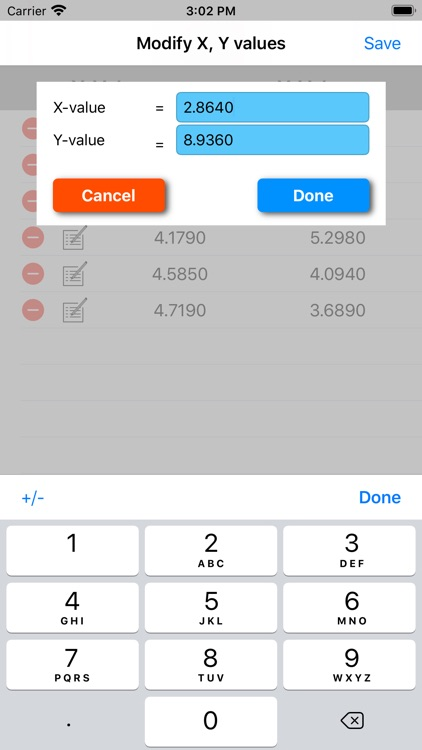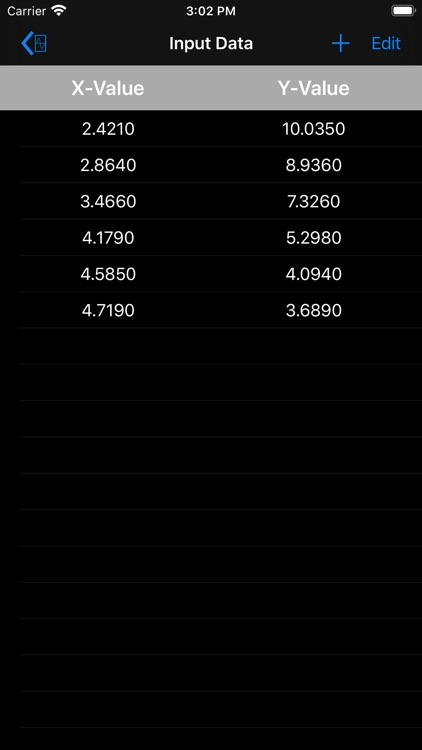### App Store Description

The Line-Fit app uses linear regression to model the relationship between two variables (x and y) by fitting a linear equation to observed data. One variable is considered to be an explanatory (independent) variable, and the other is considered to be a response (dependent) variable.

A linear regression line has an equation of the form Y = a + bX, where X is the explanatory variable and Y is the dependent variable. The slope of the line is b, and the intercept is a (the value of y when x = 0).

To measure the association between the two variables the correlation coefficient is used, which is a value between -1 and 1 indicating the strength of the association of the observed data for the two variables.

The coefficient of determination, R2, measures how close the data are to the fitted regression line. In general, higher values of R2 indicate a better fit of the model to the given data. R2 is always positive and equal to 1.0 for a perfect fit.

Disclaimer:
AppAdvice does not own this application and only provides images and links contained in the iTunes Search API, to help our users find the best apps to download. If you are the developer of this app and would like your information removed, please send a request to takedown@appadvice.com and your information will be removed.